# at

## 语法

``at(n,E)``
``at(n,tick)``
``at(n,sec)``

## 描述

`如果自关联状态激活以来 `E` 事件恰好发生了 `n` 次，则 `at(n,E)` 返回 `true`。否则，运算符返回 `false`。`

`如果图自关联状态激活以来恰好唤醒了 `n` 次，则 `at(n,tick)` 返回 `true`。否则，运算符返回 `false`。当 Simulink® 模型中的 Stateflow® 图有输入事件时，不支持隐式事件 `tick`。`

```如果自关联状态激活以来恰好经过了 `n` 秒，则 `at(n,sec)` 返回 `true`。否则，运算符返回 `false`。在 MATLAB® 中的独立图中，将 `n` 指定为大于或等于 `0.001` 的值。运算符创建一个 MATLAB `timer` 对象，该对象生成隐式事件来唤醒图。MATLAB `timer` 对象的精度限制在 1 毫秒。有关详细信息，请参阅Events in Standalone Charts。 当图完成执行关联状态及其子状态的 `entry` 动作时，将创建 `timer` 对象。如果将 `n` 指定为在图执行期间其值发生变化的表达式，则图不会调整 `timer` 对象的时滞。当关联状态激活时，`timer` 对象会在该状态图步结束时开始运行。此状态图步可以包括图中其他并行状态的执行。如果图在从 `timer` 对象接收到隐式事件时正在处理另一项操作，则图会将事件排队。当前状态图步完成后，图会处理该事件。如果与时序逻辑运算符相关联的状态在图处理隐式事件之前变为非激活状态，则该事件不会唤醒图。 注意只有 MATLAB 中的独立图支持此语法。对于 Simulink 模型中的图，请改用 `after` 运算符。有关详细信息，请参阅不要在 Simulink 模型的图中用 at 来表示绝对时间时序逻辑。 ```

## 示例

``on at(3,E): disp("ON");``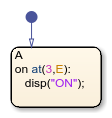`at(5,E)`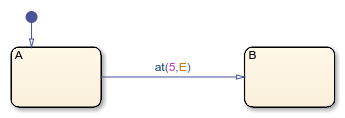`[at(5,E)]`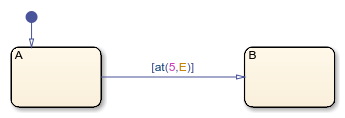MATLAB 中的独立图不支持时序逻辑运算符的条件表示法。

`at(7,tick)[temp > 98.6]`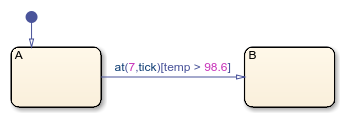``on at(12.3,sec): temp = HIGH;``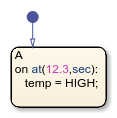## 提示

• 您可以使用引号将关键字括起来，如 `'tick'``'sec'`。例如，`at(5,'tick')` 等效于 `at(5,tick)`

• 每当关联状态重新激活时，Stateflow 图都会重置 `at` 运算符使用的计数器。

• MATLAB 中的独立图根据挂钟时间定义绝对时间时序逻辑，其精度限制在 1 毫秒。